• lc低通滤波器截止频率计算公式 LC串联时, 电路复阻抗 Z = jwL-j(1/wC) 令Im[Z]=0,即 wL=1/(wC) 得 w =根号下(1/(LC)) 此即为谐振角频率,频率自己换算. 并联时 电路复导纳 Y = 1/( jwL)+1/[-j(1/wC)]=j[wC-1/(wL)] 令...
• 对埋地充液管道的截止频率做了分析探讨，在0到截止频率之间的频率为可测量，截止频率之外的部分不可测量，并结合声场的分布规律对声音的传播做了研究
• 该软件为RC截止频率计算器、LC截止频率计算器。使用C++开发，软件界面美观，需要的可以下载。
• 所谓低通滤波器，顾名思义是指允许低频率的信号通过，而抑制高频率的信号的部件，理想的滤波器是不可能实现的。...本文讲述了如何利用传递函数计算截止频率的方法，和设计一阶、二阶、高阶低通滤波器的方法。
• 自己的电压跟随低通滤波器和无限增益低通滤波器的计算器，能计算截止频率和品质因数，电路一目了然
• ## 简单二阶滤波器截止频率的计算

万次阅读 多人点赞 2018-07-25 00:04:04
最近刚好学习到这了，而我在网上查资料的...这个电路我想大家都非常的了解，但我还是将公式推导一下 由输入电压Vi是电阻电压和电容电压的和，输出电压Vo是电容电压，所以 令Wo=1/RC 所以电压随频率的增...
最近刚好学习到这了，而我在网上查资料的时候却非常难找，不少资料讲解不够详细，所以经过我努力也为了为大家做点贡献的想法，以自己的见解写下这篇文章。废话不多说，先从一阶滤波器讲起。
一阶低通滤波器：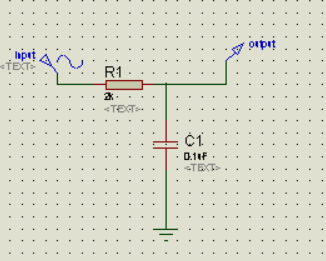这个电路我想大家都非常的了解，但我还是将公式推导一下
由输入电压Vi是电阻电压和电容电压的和，输出电压Vo是电容电压，所以令Wo=1/RC所以电压随频率的增加而减少
由截止频率时电压系数N=1/（2）0.5=0.707
即(w/w0) =1
截止频率w=w0
通过我们通过计算1/RC的值，便能计算出截止频率。当然高通滤波器也是如此，只要将输出电压换成电阻电压就行了。
接下来我们来计算二阶低通滤波器，从最简单的开始：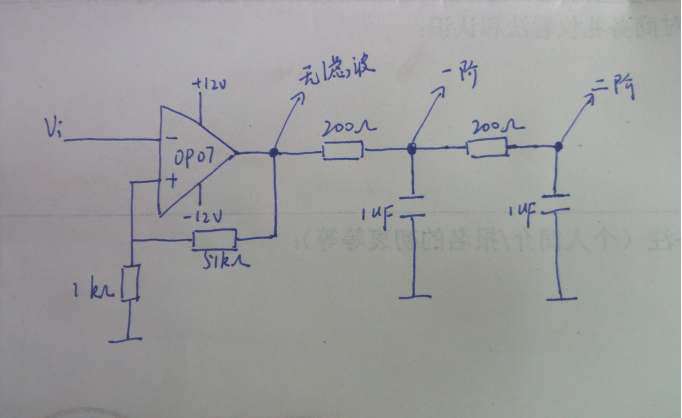这是自己画的电路：
先计算后两级的等效电阻再计算电压比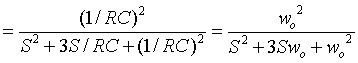通过和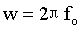最终得到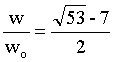展开全文• 一个电感和一个电容组成的LC谐振回路有LC串联回路和LC并联回路两种 。理想LC串联回路谐振时对外呈0阻抗，理想LC并联回路谐振时对外阻抗无穷大。利用这个特性可以用LC回路做成各种振荡电路，选频网络，滤波网络等。
• 参考模电RC滤波计算公式来计算截止频率 #define M_PI_FLOAT 3.14159265358979323846f typedef struct LpfFilter { float RC;//模电RC滤波的电阻和电容值的积 float dT;//数据更新时间隔（秒） float k;//...
参考模电RC滤波计算公式来计算截止频率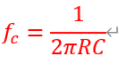#define M_PI_FLOAT  3.14159265358979323846f

typedef struct LpfFilter
{
float RC;//模电RC滤波的电阻和电容值的积
float dT;//数据更新时间隔（秒）
float k;//滤波系数
float state;//滤波结果
}LpfFilter_t;

/**设置滤波的截止频率
* *filter：
* f_cut：截止频率
* dt: 数据更新时间隔（秒）
*/
void filterInit(LpfFilter_t *filter, float f_cut, float dt)
{
filter->RC = 1.0f / ( 2.0f * M_PI_FLOAT * f_cut);//参考模电RC截止频率公式
filter->dT = dT;//数据更新时间隔（秒）
filter->k = filter->dT / (filter->RC + filter->dT);//滤波系数
}

/**应用低通滤波
* *filter：
* input：新输入数据
*/
float LpfFilterApply(pt1Filter_t *filter, float input)
{
//dt固定时，截止频率越低，k越小，数据最终输出的变化越慢（高频去掉了）
//截止频率固定时，dT越大，k越小，数据最终输出的变化越慢（高频去掉了）
filter->state = filter->state + filter->k * (input - filter->state);
return filter->state;
}
参考：
https://github.com/betaflight/betaflight/blob/master/src/main/common/filter.c
展开全文• 通过计算得到前20个截止频率为：     由上表可以看出：点数越多，计算越精确。当网格长度是波长的0.1倍时，可以计算出较为准确值。   2） FD for TE 边缘节点为虚拟节点，网格大小为a/nx-1   TE前20截止频率 ...
一、任务要求
(a) Writea finite difference (FD) code to compute the cutoff frequencies of the firsttwenty TM modes of a rectangular waveguide with dimensions 1 cm by 2 cm.Compare your results to the analytical cutoff frequencies. How rapidly do theFD results approach the exact results as the number of grid.points increases?
(b) Same as (a), but forTE modes.
(c)  Which case (TE or TM) has moreaccurate results
comparing with the analytical results? Why?

二、波导问题分析
求解波导问题相当于求解亥姆霍兹方程：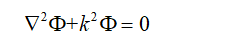（1）
上式中，对于TM模式， ，对于TE模式， ，其中 是波数。
二者边界条件为：
1）对于TM波，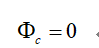2）对于TE波，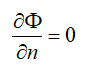在上述方程的本征值问题中， 和 都是待定，截止波长  ，对截止波长的每一个值都有一个本征函数对应，代表一个传播模式。
使用有限差分法，用正方形来剖分波导横截面得到：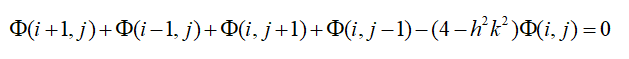(2)
式中: 为网格尺寸。
可以得到N个方程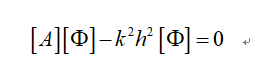求解A矩阵的特征值可得到截止波数即：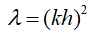。

三、matlab代码实现
1) FD for TM
对于一个20mm*10mm的波导，
网格划分图：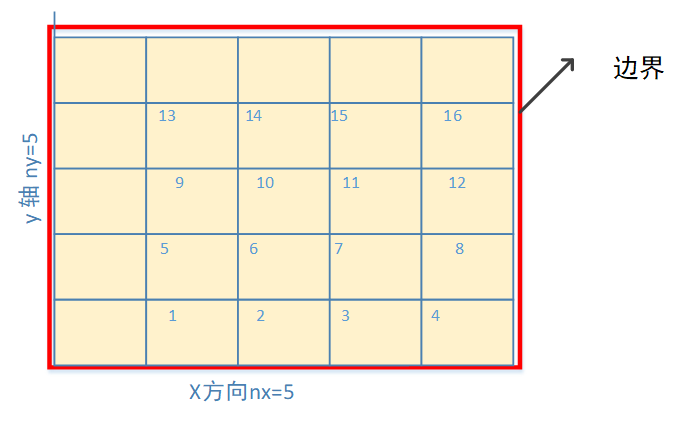边缘节点为固定矩阵，TM边界为0 ，网格大小为a/nx
通过计算得到前20个截止频率为：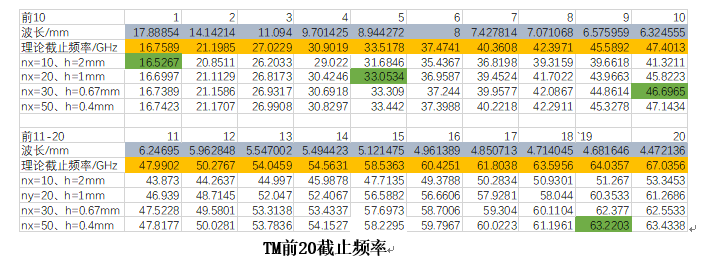由上表可以看出：点数越多，计算越精确。当网格长度是波长的0.1倍时，可以计算出较为准确值。

2）    FD for TE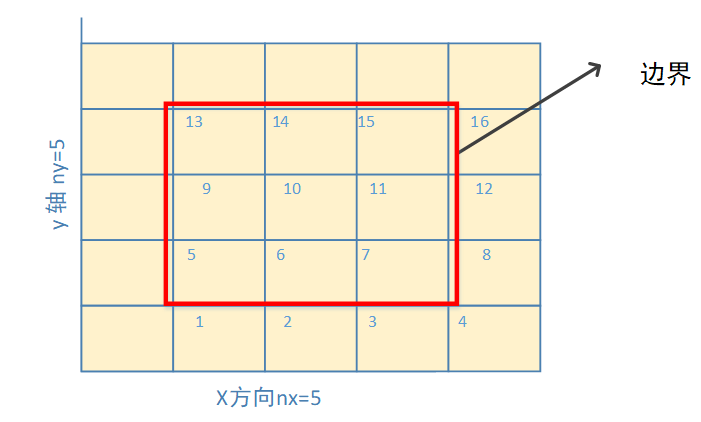边缘节点为虚拟节点，网格大小为a/nx-1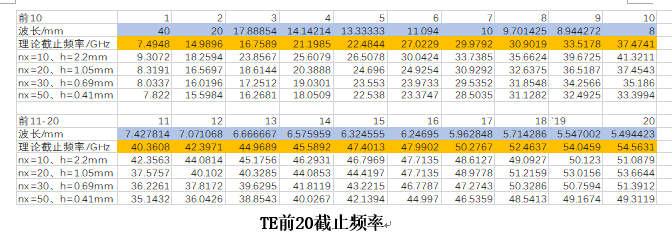TE前20截止频率
由上表可以看出：点数越多，计算越精确。但TE计算没TM较为准确，是由于在边界条件上多了一阶近似。
Matlab：代码
FD：TM

clc
clear alla=20*1e-3;         %长边20mm
b=10*1e-3;         %短边10mm
c=2.99792458*1e8;  %光速
nx=10;             %x方向剖分网格
ny=nx*0.5;         %y方向剖分网格保证为正方形
h=a/nx;            %网格大小
N=(nx-1)*(ny-1);  %未知数个数
A=zeros(N,N);
k=0 ;              %用于填充m
y1=zeros(2,20);    %前20个截止频率比较
for j=1:ny-1
for i=1:nx-1
k=k+1
NL(j,i) = k;
end             %NL用于i、j和m的对应关系
end
%%%%%填充A矩阵%%%%%%%
for m=1:N
for n=1:N
[jm,im]=find(NL==m)
[jn,in]=find(NL==n);
if m==n
A(m,n)=4;
elseif im==in&jm==jn+1
A(m,n)=-1;
elseif im==in&jm==jn-1
A(m,n)=-1;
elseif im==in+1&jm==jn
A(m,n)=-1;
elseif im==in-1&jm==jn
A(m,n)=-1;
else
A(m,n)=0;
end
end
end
%%%%%%%填充A矩阵%%%%%%%
la=eig(A);            %求特征值la
la=round(la,4);       %保留小数点后4位
la=unique(la);        %去除相同元素
Kc_fd=sqrt(la)./h;
lamda_fd=2*pi./Kc_fd;
f_fd=c./lamda_fd;
B1=f_fd;

for i=1:20
[p,q]=min(B1);     %取截止频率最小前20
B1(q)=inf;
y1(1,i)=p;         %fd
end
%%%%%%%理论计算截止频率 TM11-88 %%%%%%%%%%%
for m=1:8
for n=1:8
Kc_cal(m,n)=sqrt( (m*pi/a)^2 +(n*pi/b)^2   )
lambda_cal(m,n)=2*pi/Kc_cal(m,n)
f_cal(m,n)=c/lambda_cal(m,n);
end
end
B2=sort(f_cal(:),1,'ascend')
B2=round(B2,4)    %保留小数点后4位
B2=unique(B2)     %去除相同元素
for i=1:20
[p,q]=min(B2);
B2(q)=inf;
y1(2,i)=p;        %cal
end
y1=y1*1e-9;           %换算为GHz
y1=round(y1,4);       %保留小数点后4位
disp(y1)             % y1第一行为FD计算值    第二行为理论计算值

FD:TE
clcclear alla=20*1e-3;         %长边20mmb=10*1e-3;         %短边10mmc=2.99792458*1e8;  %光速nx=10;             %x方向剖分网格ny=nx*0.5;         %y方向剖分网格h=a/nx;            %网格大小 N=(nx-1)*(ny-1);  %未知数个数 A=zeros(N,N);       k=0 ;              %用于填充m y1=zeros(2,20);    %前20个截止频率比较  for j=1:ny-1      for i=1:nx-1         k=k+1        NL(j,i) = k;      end             %NL用于i、j和m的对应关系  end  %%%%%填充A矩阵%%%%%%%  for m=1:N      for n=1:N  [jm,im]=find(NL==m)  [jn,in]=find(NL==n);  if m==n           A(m,n)=4;  elseif im==in&jm==jn+1        A(m,n)=-1;  elseif im==in&jm==jn-1        A(m,n)=-1;  elseif im==in+1&jm==jn        A(m,n)=-1;  elseif im==in-1&jm==jn        A(m,n)=-1;   else           A(m,n)=0;  end    %%%%%%%%%%%  TE边界条件 %%%%%%%%%     if im==in&jm==jn-1&jm==1                %下边界         A(m,n)=-2;   elseif im==in&jm==jn+1&jm==(ny-1)        %上边界         A(m,n)=-2;   elseif im==in-1&jm==jn& im==1            %左边界         A(m,n)=-2;   elseif im==in+1&jm==jn&im==(nx-1)        %右边界         A(m,n)=-2;   end endend %%%%%%%填充A矩阵%%%%%%%  la=eig(A);            %求特征值la  la=round(la,4);       %保留小数点后4位  la=unique(la);        %去除相同元素  Kc_fd=sqrt(la)./h;     lamda_fd=2*pi./Kc_fd;  f_fd=c./lamda_fd;  B1=f_fd;             B1(find(B1==0))=[];    %去除0for i=1:20    [p,q]=min(B1);     %取截止频率最小前20    B1(q)=inf;    y1(1,i)=p;         %fdend%%%%%%%理论计算截止频率 TE10- TE87 %%%%%%%%%%%for m=1:8   for n=1:8Kc_cal(m,n)=sqrt( (m*pi/a)^2 +((n-1)*pi/b)^2   )lambda_cal(m,n)=2*pi/Kc_cal(m,n)f_cal(m,n)=c/lambda_cal(m,n);   endendB2=sort(f_cal(:),1,'ascend')B2=round(B2,4)    %保留小数点后4位B2=unique(B2)     %去除相同元素for i=1:20    [p,q]=min(B2);    B2(q)=inf;    y1(2,i)=p;        %calendy1=y1*1e-9;           %换算为GHzy1=round(y1,4);       %保留小数点后4位disp(y1)  % y1第一行为FD计算值    第二行为理论计算值    新人贴，好紧张呐


展开全文matlab 差分法 矩形波导
• ## 带宽与截止频率

千次阅读 2021-03-10 14:37:31
带宽与截止频率 https://blog.csdn.net/a419116194/article/details/103335162 http://m.elecfans.com/article/588901.html 一、带宽，信号频率概念解释 带宽：信号带宽是信号频谱的宽度，信号波是由n个正弦波...
带宽与截止频率
https://blog.csdn.net/a419116194/article/details/103335162
http://m.elecfans.com/article/588901.html
一、带宽，信号频率概念解释
带宽： 信号带宽是信号频谱的宽度，信号波是由n个正弦波叠加而成的，那么信号的带宽也就是着n个谐波信号中的最高频率分量与最低频率分量之差。信号频率： 由信号频谱图可以观察到一个信号所包含的频率成分。把一个信号所包含谐波的最高频率与最低频率之差，即该信号所拥有的频率范围，定义为该信号的带宽，信号的频率变化范围越大，信号的带宽就越宽。 例如：一个由n个正弦波叠加成的方波信号，其最低频率分量是其基频，假定为f=2kHz，其最高频率分量是其7次谐波频率，即7f =7×2=14kHz，因此该信号带宽为7f - f =14-2=12kHz。信道带宽限定了允许通过该信道的信号下限频率和上限频率，也就是限定了一个频率通带。 例如：一个信道允许的通带为1.5kHz至15kHz，其带宽为13.5kHz，上面这个方波信号的所有频率成分当然能从该信道通过，如果不考虑衰减、时延以及噪声等因素，通过此信道的该信号会毫不失真。 然而，如果一个基频为1kHz的方波，通过该信道肯定失真会很严重；方波信号若基频为2kHz，但最高谐波频率为18kHz，带宽超出了信道带宽，其高次谐波也会被信道滤除，通过该信道接收到的方波没有发送的质量好；
二、截止频率
低通滤波电路 　　低通滤波器是指车载功放中能够让低频信号通过而不让中、高频信号通过的电路，其作用是滤去音频信号中的中音和高音成分，增强低音成分以驱动扬声器的低音单元。此外高通滤波器常常和低通滤波器成对出现，不论哪一种，都是为了把一定的声音频率送到应该去的单元。 　　特点：低损耗高抑制；分割点准确；双铜管保护；频蔽好防水功能强。 　　用途：产品用途广泛，使用于很多通讯系统，如 CATV EOC 等系统。并能有效的除掉通频带以外的信号和多余的频段、频率的干扰。
下图所示为10MHz低通滤波器，此低通滤波器利用带宽高达100MHz的高速电流反馈集成运放0PA603组成二阶巴特沃思低通滤波器滩图中R1＝R2＝159Ω ，C1＝C2=100pF，其截止频率为fc=1/2πR1C1=10MHz，其零频增益为G0＝1+Rf/R＝1.6。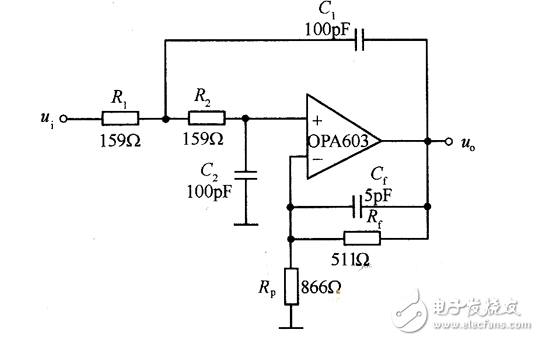截止频率 当保持输入信号的幅度不变，改变频率使输出信号降至最大值的0.707倍，即用频响特性来表述即为-3dB点处即为截止频率，它是用来说明频率特性指标的一个特殊频率。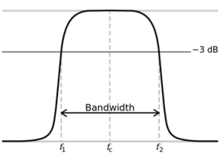截止频率计算方法 利用系统函数的模来表示电路的放大倍数，由于20lgA（ω）=-3dB，解得:A（ω）=10-0.15=0.707945784≈1/√2，又因为A（ω）=|H（jω）|，则|H（jω）|^2=1/2 在高频端和低频端各有一个截止频率，分别称为上截止频率和下截止频率。两个截止频率之间的频率范围称为通频带。
开环截止频率与闭环截止频率 　　开环截止频率也称为剪切频率，是开环幅频特性中，幅频特性曲线穿越0dB线的频率，记为ωc；闭环截止频率也称为带宽频率，是指当闭环幅频特性下降到频率为零时的分贝值以下3dB时，对应的频率，记作ωb。 　　开环截止频率与闭环截止频率具有同向性。开环截止频率与闭环截止频率是两个完成不同的物理量，分别用于描述开环系统和闭环系统的幅频特性，但它们之间又存在一定的相关性，即：开闭截止频率与其单位负反馈的闭环截止频率是同向增大的。且具有如下关系：ωb>ωc。 　　由于闭环截止频率可用来表征闭环系统瞬态响应速度，闭环截止频率ωb越高，其瞬态响应速度越快。既然ωc与ωb具有同向性，则可通过系统的Bode图就可知道系统的瞬态响应速度，即剪切频率ωc越高，瞬态响应速度越快。
低通滤波器带宽和截止频率的关系 　　带宽B一般用截止频率f=ωH/2π来定义，B=1/f=2π/ωH，也可以理解为B带宽其实就是上下截止频率的差 　　实际应用中，对于信号的负频率部分，需要经过希尔伯特变换器，剔除负频率部分，这样可以在信号调制后有效节约带宽。 　　低通滤波器带宽就等于截止频率，高通滤波器一般很少说带宽，只说截止频率。带通滤波器带宽和截止频率才有明显区别

二阶低通滤波器截止频率公式为fc=1/（2pi（R1R2C1*C2）^.5）

假如是下面这个电路，可以实现方波转换成正弦波吗？假如我要把1KHz~100KHz的方波信号转换成正弦波信号，又该怎样改变R、C的大小？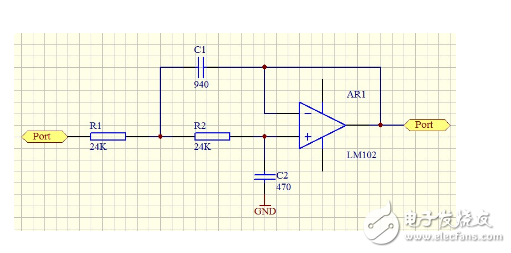想把1kHz方波转换成正弦波可以用这个电路，只要把截止频率设置在1kHz上即可；想把100kHz方波转成正弦波也可以用它，但是要重调截止频率。要把1~100kHz范围转换，这个电路做不到，因为能通过100kHz正弦波的低通，对1kHz的方波畅通无阻（这个方波内包含的3、5、7、9次。。。等等谐波分量均在100kHz以内）。
改变通带频率只要改变C1、C2，容量增加几倍，频率就下降几倍，反之亦然。当然不改电容，改变R1、R2也行，结果类同。没有工程师自己计算截止频率，都是用查手册的方法解决问题；对于你这个现成电路，还可以用软件仿真测量出频响。
怎样设计电路来实现1~100KHz任意频率的波形转换呢？
1、硬件电路：用函数信号芯片8038，直接出方波、三角波、正弦波三种波形。核心是一个三角波振荡器，其中正弦波是对三角波进行“断点整形”而来（用方波整不出来），而方波是用过上下双门限比较器获得。 2、软件电路：把正弦波函数表存入ROM，反复读取，通过D/A输出。
展开全文• 最基础的滤波器是由电阻和电容构建的RC滤波器，有低通和高通滤波器之分，RC滤波器的截止频率计算公式为：F(cutoff) = 1 / (2πRC)。截止频率，就是滤波器频率响应出现拐点的频率。一、RC低通滤波器构建RC低通...
• 在自动控制原理课程中，利用折线式伯德图计算截止频率是很常见的题型，下面介绍两种做法。 对于以下传递函数： G(s)=50s2(s2+s+1)(10s+1)=G1(s)G2(s)G3(s)G4(s)G5(s){G(s)=\frac{50}{s^2(s^2+s+1)(10s+1)}=G_1(s)G_2...
• 2.求截止频率 （1）求谐振频率 谐振时，感抗等于容抗，此时电路对外呈现纯电阻特性，同时由上式可知，此时电路电流最大，由XL=XC可求出谐振频率f0。 （2）求品质因素 因为谐振时，所以品质因素可由以上两种...
• 按照时域采样定理，采样频率≥2倍的信号频率，才能得到信号全部信息。而以智能车中的编码器测速为例。我们知道测速周期在可接受范围内越小越利于控速，比如2ms。但2ms采样一次速度，究竟能不能得到速度信号的全部...
• 截止波数 kck_ckc​ kc=(mπa)2+(nπb)2k_c=\sqrt{(\frac{m\pi}{a})^2+(\frac{n\pi}{b})^2}kc​=(amπ​)2+(bnπ​)2​ 截止波长 λc\lambda_cλc​ λc=2πkc=2(ma)2+(nb)2\lambda_c=\frac{2\pi...截止频率 fcf_cfc​.
• ## 关于-3db截止频率

万次阅读 多人点赞 2018-05-23 15:02:17
电流，电压等中 10*lgX=1dB; 功率中 为20*lgX = 1dB.区别只是表示是功率还是电流等啦。功率都是20*lgX.其他10*lgX. ...关于-3db截止频率为什么当信号衰减了-3db的时候就算是截止频率了。这里面...
• 对差分法所获的离散差分公式作矩阵变换,使差分离散方程的系数矩阵对于波导的各阶模都是正定的,从而可利用该变形离散方程计算波导各个高阶模的截止频率。编程实例计算了矩形波导、L形波导、对称脊波导部分高阶模的...
• 使用二阶高通有源滤波器.exe软件过程中，先在编辑框中输入电阻R1、Rf、R、C的值。电阻、电容的数量级可以分别通过下拉列表框进行选择。R1、Rf、R、C如图二阶高通有源滤波器电路图.bmp所示，谢谢使用！
• 原文链接：http://www.elecfans.com/tools/rclvboqijiezhipinlv.html 点击打开链接
• 最近在设计方案时，遇到了有关滤波器的问题，好难搞定哦；所以就顺便总结下滤波器的基础知识。...什么是滤波器滤波器是指一个电路，它能够去除掉人为指定的某个频率范围的信号分量；因为模拟信号...
• 尽管滤波器会消除信号模糊，但它同样会阻止任何频率高于滤波器的截止频率的其它信号通过滤波器，不管这些信号是需要的还是不需要的。也就是说，当选择一个数据采集系统的时候，务必确定每个通道的采样频率高于两倍的...
• 低通滤波器传输函数极大值为： 由∣H(Ω)∣=0.707∣H(Ω)∣max可以求得低通滤波器上限截止频率，结果表明，对于低通滤波器．仍为α越大，低通滤波器上限频率(即带宽)越小。 该内容是小编转载自网络，仅供学习交流...
• 在之前的文章中，我们看到系统的频率响应可以用极坐标图表示...截止频率的重要性上图不包括一条非常重要的信息，即滤波器的截止频率。一阶低通滤波器的s域传递函数可表示如下：这个公式告诉我们，一个给定的低通滤波...
• 1、中心频率对应的时间常数转折频率相同的高、低通滤波器合成带通电路，高低通的转折...移项得电阻电容计算式R=1/2fcC和C=1/2fcR。例如中心频率fc=100KHz，则R=1.592K，C=1nf。2、通频带对应的时间常数fc=fo=...
• 二、滤波器电路图及其截止频率计算公式 一阶RC滤波器电路图如上，截止频率公式为：f=1/(2πRC) 如R=51Ω，C=22uF，则截止频率f=141Hz。意味着，高通RC滤波器会对低于141Hz的信号起抑制作用。低通滤波器会对...
• 采用矢量场法,得到了在弱导条件下传导模式的特征方程和截止频率的近似计算公式。引入了介电比γ,它是由光纤三个折射率参量所确定的常数。在弱导近似的条件下,归一化截止频率仅与γ这一个变量有关,任一渐变抛物型光纤......